Electron. J. Differential Equations, Vol. 2017 (2017), No. 115, pp. 1-13.

### Existence of solutions for degenerate Kirchhoff type problems with fractional p-Laplacian Nemat Nyamoradi, Lahib Ibrahim Zaidan

Abstract:
In this article, by using the Fountain theorem and Mountain pass theorem in critical point theory without Palais-Smale (PS) condition, we show the existence and multiplicity of solutions to the degenerate Kirchhoff type problem with the fractional p-Laplacian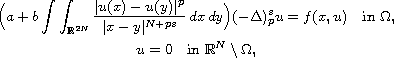where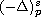is the fractional p-Laplace operator with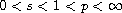,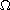is a smooth bounded domain of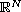, N > 2 s, a, b > 0 are constants and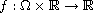is a continuous function.

Submitted February 23, 2017. Published April 27, 2017.
Math Subject Classifications: 34B27, 35J60, 35B05.
Key Words: Kirchhoff nonlocal operators; fractional differential equations; fountain theorem; mountain pass theorem; critical point theory.

Show me the PDF file (281 KB), TEX file for this article.Nemat Nyamoradi Department of Mathematics Faculty of Sciences, Razi University 67149 Kermanshah, Iran email: nyamoradi@razi.ac.ir, neamat80@yahoo.com Lahib Ibrahim Zaidan Department of Mathematics, Faculty of Sciences, Razi University 67149 Kermanshah, Iran email: lahibzaidan75@yahoo.com; lahibzaidan@uobabylon.edu.iq